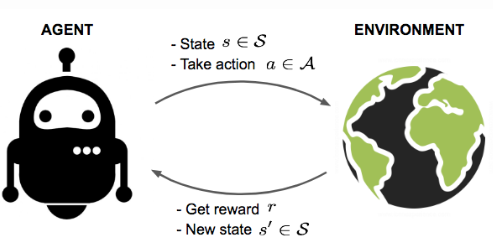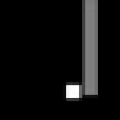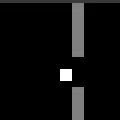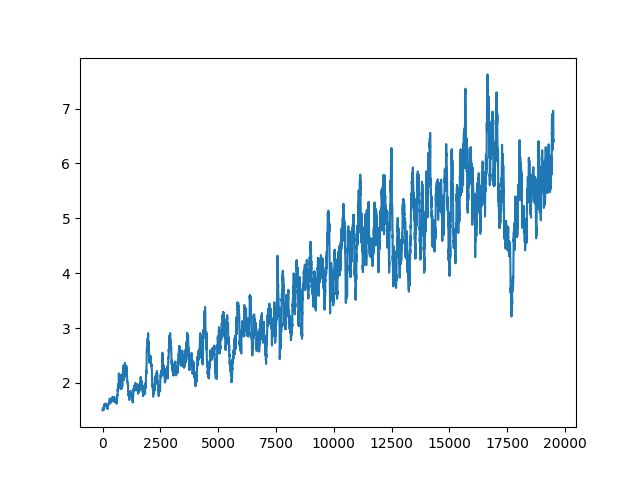Reinforcement Learning (RL) has had tremendous success in many disciplines of Machine Learning. While the results of RL almost look magical, it is surprisingly easy to get a grasp of the basic idea behind RL. In this post, we will discuss the basic principles of RL and we will implement a simple environment in which an agent tries to stay alive as long as possible by means of RL.

We will use the previously discussed Concept-Math-Code (C-M-C) approach to gain drive our process of understanding RL.

## Concept

Broadly speaking, Reinforcement Learning allows an autonomous agent to learn to make intelligent choices about how it should interact with its environment in order to maximize a reward. The environment can be as simple as a single number expressing a measurement the agent takes, or it can be as complex as a screenshot of the game DOTA2, which the agent learns to play (see https://openai.com/).

For every discrete time step, the agent perceives the state $$s$$ of his environment and chooses an action $$a$$ according to its policy. The agent then receives a reward $$r$$ for its action and the environment transitioned into the next state $$s’$$.The feedback loop of RL (image credit: )

In order to make RL work, the agent does not necessarily need to know the inner workings of the environment (i.e. it does not need a model of its environment predicting future states of the environment based on the current state). However, learning speed increases if the agent incorporates as much knowledge about the environment a priori as possible.

## Q-Learning

Q-Learning was a big breakout in the early days of Reinforcement-Learning. The idea behind Q-Learning is to assign each Action-State pair a value — the Q-value — quantifying an estimate of the amount of reward we might get when we perform a certain action when the environment is in a certain state. So, if we are in a state S, we just pick the action that has the highest assigned Q-value as we assume that we receive the highest reward in return. Once we performed action a, the environment is in a new state S’ and we can measure the reward we actually received in return for performing action a. Once we measured the reward for performing action a, we can then update the Q-values of the Action-Space pair since we now know which rewards we actually received by the environment for performing action a. How the Q-values are actually updated after every time step, we will discuss in the Math section this post.

You might already have noticed a wide-open gap in the Q-Learning algorithm: How the heck are we supposed to know the Q-values of a state-action pair? We might consider updating a table in which we save the Q-values of each state-action pair. Every time we take an action in the environment, we store a new Q-value for a state-action pair. These actions do at first not even have to make sense or lead to high rewards since we are only interested in building up a table of Q-values which we can use to make more intelligent decisions later on. But what if the state S in which an environment has high dimensionality or is sampled from a continuous space? We cannot expect our computer to store infinite amounts of data. What can we do? Neural Networks to the rescue!

## Deep Q-Learning

Instead of updating a possibly infinite table of state-action pairs and their respective Q-values, let’s use a Deep Neural Network to map a state-action pair to a Q-value, hence the name Deep Q-Learning.

If the network is trained sufficiently well, it is able to tell with high confidence what Q-values certain actions might have given a state S in which the environment currently is. While this sounds super easy and fun, this approach suffers from instability issues and divergence. Two main mechanisms were introduced by Mnih et al. in 2015 in order to avoid these issues: Experience Replay and Frozen Optimization Target. Briefly stated, experience replay allows the network to learn on a single experience $$e_{t+1}$$ consisting of a state $$s_t$$, an action $$a_t$$, reward $$r_t$$, and new state $$s_{t+1}$$ tuple more than one time. The term “frozen optimization target”, in contrast, refers to the fact that the Q-value estimation network used for predicting future Q-values is not the same as the network used for training. Every $$N$$ steps, the values of the trained network are copied to the network being used to predict future Q-values. It was found that this procedure leads to much less instability issues during training.

# Math

We have established the concepts behind Q-Learning and why Deep Q-Learning solves the issue with storing possibly infinite numbers of state-action pairs. Let us now briefly dive into some of the math involved in Deep Q Learning.

During the training of the neural network, the Q-values of each state-value pair is updated using the following equation:

\begin{align*} Q(S_{t+1}, A_{t+1}) = Q(S_t, A_t) + \alpha [R_{t+1} + \gamma \max_{a \in A} Q(S_{t+1}, a) - Q(S_t, A_t) ] \end{align*}

Let us first discuss the term in square brackets. The variable $$R_{t+1}$$ denotes the reward given to the agent at time step $$t+1$$. The next addend denotes the maximal Q-value for all possible actions a while the environment is in the new state $$S_{t+1}$$. Beware that this value can also only be an estimate of the true maximal Q-value as we can only estimate future Q-values of state-action pairs. This maximal Q-value is multiplied by a so-called discount factor (denoted gamma). This factor decides how much weight we assign to future rewards in comparison to the currently achieved reward. If the discount factor equals zero, only the current reward matters to the agent, and no future rewards matter for estimating future Q-values. The discount factor is typically set to a value of about $$0.95$$. The last addend in the square brackets is simply the current Q-value estimate again. Thus, the term in the square brackets as a whole expresses the difference between the predicted Q-value and our best estimate for the true Q-value. Bear in mind that obviously also our best estimate for the true Q-value might not be totally perfect since we only know the reward for the next time step $$R_{t+1}$$ for sure, but this little bit of knowledge helps us to improve the Q-value estimate for the current time step.

This whole difference-term in square brackets is multiplied by the learning rate alpha that weighs how much we trust this estimated error between the best estimate of the true Q-value and the predicted Q-value. The bigger we choose alpha, the more we trust this estimate. The learning rate is typically between $$0.01$$ and $$0.0001$$.

With our understanding of the Q-value update, the loss of a Q-value prediction can be described as follows:

\begin{align*} \mathcal{L} (\theta) = E_{(s,a,r,s') \sim U(D)} \Big[ \big(r + \gamma \max_{a'} Q(s', a'; \theta^{-}) - Q(s, a; \theta) \big)^2 \Big] \end{align*}

Do not let yourself be intimidated by the term before the brackets on the right-hand-side of the equation. This part basically means that we randomly sample a state-action-reward-new_state $$(s,a,r,s’)$$ tuple from the replay memory called $$D$$. The term within the square brackets defines the mean squared error between the actually observed reward r added to all expected future rewards beginning from the next time step (including the discount factor gamma) AND the actually by the neural network predicted Q-value. Pretty simple, right? During training, the prediction of the Q-values should become increasingly better (however, strong fluctuations during learning do usually happen).

These already are the most important bits of math in Deep Q-Learning. We could of course discuss some of the beauty of Linear Algebra and Calculus involved in the Neural Network estimating the Q-values. However, this is beyond the scope of this post and smarter people have done a much better job at explaining this (i.e. the truly awesome 3Blue1Brown).

# Problem statement

We consider an agent trying to survive in a flappy-bird-like environment. The agent has to find the hole in a moving wall coming its way in order to survive. The goal is to survive as long as possible (sorry, dear agent, but there is no happy end for you). For every time-step the agent receives a reward of 0.1. When the agent learns to maximize its reward, it consequently learns to survive as long as possible and find the holes in the moving walls.White agent and grey moving walls (hard to see in this static image)

The action space consists of three possible actions: {Move up, move down, stay}. The environment consists of an 80-by-80 grey-scale pixel grid. The agent is indicated in grey color, the moving walls are indicated with white color. The agent is supposed to learn optimal behavior by just looking at the raw pixels without any further knowledge about the world. This approach is both the slowest to learn but also the most general approach since no rules have to be hard-coded into the learning system (model-free Reinforcement Learning). During training, the network learns to map the information of the raw pixels of the environment to the Q-values of all three possible actions. The policy we implemented always selects the action with the highest associated Q-value.

# Code

Without further ado, let’s have a look at the code. All relevant bits are (hopefully) commented well enough.

## Environment

We could use one of the many pre-defined environments that come with an installation of the OpenAI gym Python package. However, we could also quickly write our own small environment. As already mentioned, I implemented a crappy Flappy Bird clone featuring a square-shaped robot trying to avoid the oncoming walls.

import gym
from gym import spaces
import numpy as np
from gym import utils
from random import randint

class Obstacle:

def __init__(self):
self.hole_top = randint(0, 30)
self.hole_bottom = self.hole_top + 10
self.pos_x = 40

def reset(self):
self.hole_top = randint(0, 30)
self.hole_bottom = self.hole_top + 10
self.pos_x = 40

def step(self):
self.pos_x -= 1  # increment position
if self.pos_x < 0:  # reset obstacle if outside environment
self.reset()

def set_pos_x(self, pos_x):
self.pos_x = pos_x

def get_pos(self):
return self.pos_x

def get_hole(self):
return self.hole_top, self.hole_bottom

class Robot:

def __init__(self):
self.height = 0

def move(self, direction):
if direction == 0 and self.height > 0:
self.height -= 2  # move up
if direction == 1 and self.height < 40-5:
self.height += 2  # move down
if direction == 2:
self.height = self.height  # stay

def set_height(self, height):
self.height = height

def get_height(self):
return self.height

def get_x(self):
return 20

def reset(self):
self.height = randint(5, 35)

def __init__(self):

from gym.envs.classic_control import rendering

self.viewer = rendering.SimpleImageViewer()

self._action_set = {0, 1}  # go up, go down
self.action_space = spaces.Discrete(len(self._action_set))

# init obstacle
self.obstacle = Obstacle()

# init robot
self.robby = Robot()

# if game is over, it resets itself
def reset_game(self):

self.robby.reset()
self.obstacle.reset()

# a single time step in the environment
def step(self, a):

reward, game_over = self.act(a)
ob = self._get_obs()
info = {}

return ob, reward, game_over, info

# perform action a
def act(self, a):

self.obstacle.step()
self.robby.move(a)

rob_pos_y = self.robby.get_height()
rob_pos_x = self.robby.get_x()

top, bottom = self.obstacle.get_hole()
obstacle_pos_x = self.obstacle.get_pos()

distance_x = abs(rob_pos_x - obstacle_pos_x)

collide_x = distance_x < 5
collide_y = rob_pos_y < top or (rob_pos_y + 5 > bottom)

game_over = False
reward = 0.0

if collide_x and collide_y:
game_over = True
else:
reward = 0.1

return reward, game_over

@property
def _n_actions(self):
return len(self._action_set)

def reset(self):
self.reset_game()
return self._get_obs()

def _get_obs(self):
img = self._get_image

# image must be expanded along first dimension for keras
return np.expand_dims(img, axis=0)

def render(self):

img = self._get_image

# image must be expanded to 3 color channels to properly show the content
img = np.repeat(img, 3, axis=2)

# show frame on display
self.viewer.imshow(img)

return self.viewer.isopen

@property
def _get_image(self):

img = np.zeros(shape=(40, 40, 1), dtype=np.uint8)

obstacle_x = self.obstacle.get_pos()
width = 4

img[:, obstacle_x:obstacle_x + width, 0] = 128

top, bottom = self.obstacle.get_hole()

img[top:bottom, obstacle_x:obstacle_x + width, 0] = 0

rob_y = self.robby.get_height()
rob_x = self.robby.get_x()

img[rob_y:rob_y + width, rob_x:rob_x + width, 0] = 255

return img

def close(self):
if self.viewer is not None:
self.viewer.close()
self.viewer = None


## Agent

import random
import numpy as np
from tensorflow.keras.models import Sequential
from tensorflow.keras.layers import Dense, Conv2D, Activation, Flatten
from tensorflow.keras.models import model_from_yaml
from collections import deque

class DQNAgent:
def __init__(self, state_size, action_size, model_dir=None):

self.state_size = state_size
self.action_size = action_size
self.memory = deque(maxlen=2000)
self.gamma = 0.95  # discount rate
self.epsilon = 1.0  # exploration rate
self.epsilon_min = 0.01
self.epsilon_decay = 0.995
self.learning_rate = 0.001

if model_dir:
else:
# creating model from scratch
self.model = self._build_model()

def _build_model(self):

seqmodel = Sequential()
seqmodel.add(Conv2D(32, (8, 8), strides=(4, 4), input_shape=(40, 40, 1)))

return seqmodel

def remember(self, state, action, reward, next_state, done):

# store S-A-R-S in replay memory
self.memory.append((state, action, reward, next_state, done))

def act(self, state):
if np.random.rand() <= self.epsilon:
return random.randrange(self.action_size)
act_values = self.model.predict(state)

action = np.argmax(act_values)

return action

def replay(self, batch_size):

minibatch = random.sample(self.memory, batch_size)
for state, action, reward, next_state, done in minibatch:
target = reward
if not done:
target = (reward + self.gamma *
np.amax(self.model.predict(next_state)))
target_f = self.model.predict(state)
target_f[action] = target

# do the learning
self.model.fit(state, target_f, epochs=1, verbose=0)

if self.epsilon > self.epsilon_min:
self.epsilon *= self.epsilon_decay



## Training loop

import time
import numpy as np
from collections import deque
from DQNAgent import DQNAgent

# Initialize environment

# size of input image
state_size = 80 * 80 * 1

# size of possible actions
action_size = env.action_space.n

# Deep-Q-Learning agent
agent = DQNAgent(state_size, action_size)

# How many time steps will be analyzed during replay?
batch_size = 32

# How many time steps should one episode contain at most?
max_steps = 500

# Total number of episodes for training
n_episodes = 20000

scores_deque = deque()
deque_length = 100
all_avg_scores = []

training = True

for e in range(n_episodes):

state = env.reset()
reward = 0.0

start = time.time()

for step in range(max_steps):

done = False

action = agent.act(state)
next_state, reward_step, done, _ = env.step(action)
reward += reward_step
agent.remember(state, action, reward, next_state, done)
state = next_state

if done:
scores_deque.append(reward)
if len(scores_deque) > deque_length:
scores_deque.popleft()

scores_average = np.array(scores_deque).mean()
all_avg_scores.append(scores_average)

print("episode: {}/{}, #steps: {},reward: {}, e: {}, scores average = {}"
.format(e, n_episodes, step, reward, agent.epsilon, scores_average))
break

if training:
if len(agent.memory) > batch_size:
agent.replay(batch_size)


As always, you may find the complete code in the project GitHub repository.

# Results

Let us look at one episode of the agent playing without any training:Random actions of untrained agent (sorry about the GIF artefacts)

The agent selects actions at random as it cannot yet correlate the pixel grid with appropriate Q-values of the agent’s actions.

Pretty bad performance, I would say. How well does the agent perform after playing 20000 episodes? Let’s have a look:**

We begin to see intelligent behavior. The agent steers towards the holes once it is close enough. However, even after several thousands of episodes, the agent sooner or later crashes into one of the moving walls. It might be necessary to increase the number of training episodes to 100,000.

## Averaged reward plot

The averaged reward plot helps us to understand the training progress of the agent.Rolling average of rewards plotted over the episode number

A clear upward trend of the rolling average of the reward can be made out. Strong fluctuations of rewards are a typical observation in Reinforcement Learning. Other environments may lead to even stronger fluctuations, so do not let yourself be crushed if your rewards do not seem increase during training. Just wait a little longer!

That’s all I wanted to talk about for today. Please find the following exemplary resources if you want to dive deeper into the topic of Reinforcement Learning: